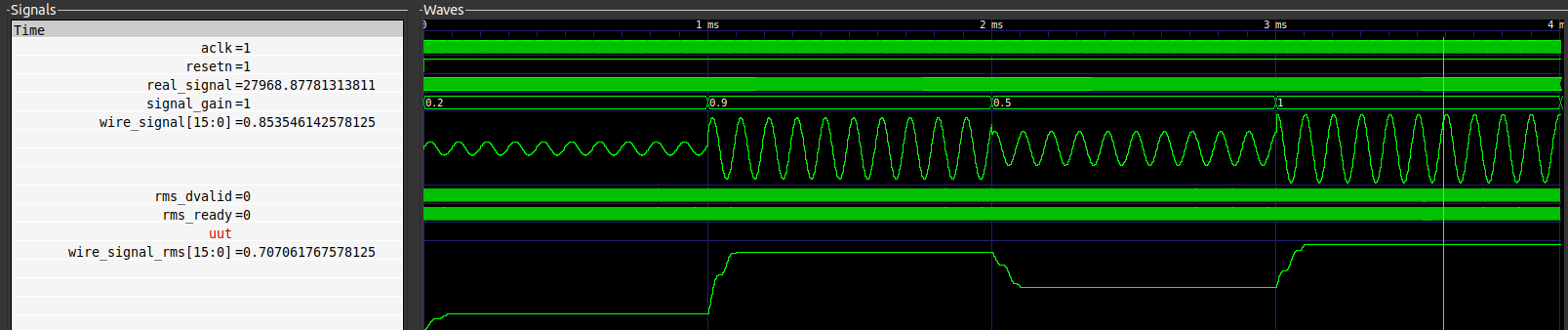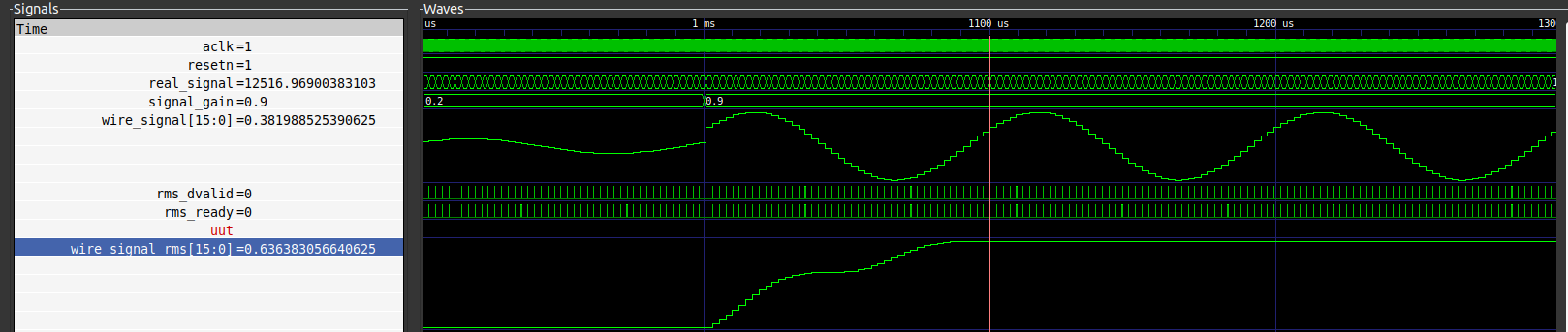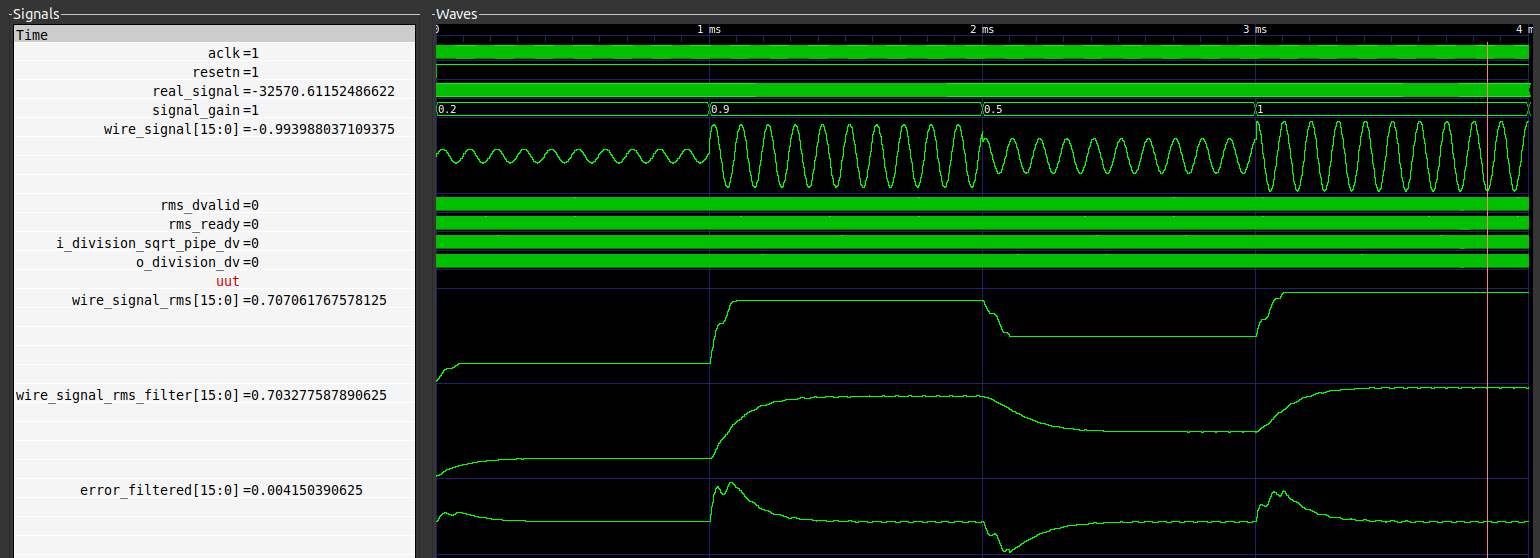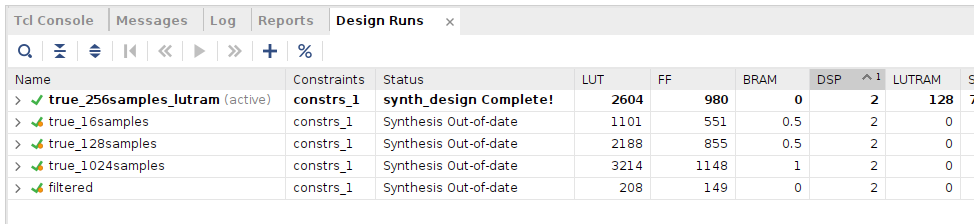When we are designing an acquisition system, sometimes process the signal is not enough, there are many cases where the project requires that the value of a measurement must be shown in a user interface. This is very easy when we are working with DC signals, since we can send to the display the value of each reading made by the ADC, and, depending of the level of noise, the measure can be valid, but the thing is very different if we have a noisy signal, or an AC signal. In this cases, sending the acquired value of the ADC is quite unuseful, because the value of the reding will be erratic. Also, notice that the sampling frequency of the visualization systems could be low, so we will have some undesirable effects in the visualization like aliasing. The solution for DC noisy signals is simply to filter the signal in order to obtain the mean of the signal. In case of the AC measurements the thing changes. Obtain the mean value of an AC signal will return a value of zero, so we will loss all the information of the signal. For these cases, we have other measurement that we can use to obtain the level of the signal, the root mean square (RMS).

RMS value, as we can read, is the square root of the mean of the square values. The fact that square the values of the samples will allow us to obtain a measurement of AC signals, since the signal is “rectified”. If we perform this steps, we say that we are calculating the true RMS.

$RMS=\sqrt{\frac{\sum_{n=0}^{N-1}{x_n^2}}{N}}$

The RMS value in AC signals also has the advantage that we can work with periodic AC signals as they are DC signals to obtain some measurements, for example, to obtain the dissipated power in a resistor that we apply an AC signal, we can apply the ohm’s law using the RMS of the voltage. For inductors and capacitors it is a bit different since we have to work with complex numbers.

$I_{RMS}=\frac{V_{RMS}}{R}$

The RMS is widely used in audio and also in grid connected devices, since the grid voltage of our houses is AC, so the values of voltage and current are given in RMS, that is used to obtain a power of a certain devices.

The calculation of the RMS is not as simple as the mean calculation, of even the filering, since it involves an addition of squares, a division and also an square root. In addition, if we talk about implement a RMS calculation in an FPGA the thing seems that it turns dark. First of all, the additions of the squares is not a big deal, we have mutiplicators in FPGA so we only have to square the signal and accumulate the result. Then, the division, that is an operation that we already see in this blog, the key in this case is that the numerator is an accumulator which width will depend of the number of samples we take, but it is only a time issue, and finally, the square root. IN the blog we have seen how, using CORDIC, and the hyperbolic kernel, we can calculate the square root, but this method has a limitation in the operation range. Using CORDIC to perform a square root only allow a precise result when the radicand is in the range from 0.5 to 2. This can be workarounded by normalising the radicand to this range and then undoing the normalization. In this ocasion I am going to use other algorithm to compute the square root, the non-restoring square root algorithm. We will talk about it later.

First of all, lets talk about the addition of the squares. Before begin to write code, we need to think how we want to work our RMS computation. Do we want a continous calculation? Is eanough for the application obtain a result after a number of cycles? The answers for these two questions will determine the way that we will perform the addition of the squares.In the case of this post I have developed a continous update RMS compute, so we will obtain a new RMS value after each sample is acquired. To do that we need to calculate the mean of the squares at sampling time. Because we are efficient engineers, we are not going to perform the addition of all the samples when a new sample arrives, instead of that, we are going to add the new sample to the accumulator, and subtact the older one. Using this we only have to perform a subtraction and an addition at the sampling frequency.

$acc = \sum_{n=0}^{N-1}{x_n^2} = acc+x²-x²_n$

In addition, this method alow us to make that in two clock cycles using RAM blocks instead of LUTRAM. I am going to explain that. If we need to perform an addition of 16 samples, we can store the 16 samples in 16 registers (in Xilinx we do that using the instruction (* ram_style = 'distributed' *) in the matrix declaration). This way we have the 16 samples accesible in one clock cycle. Although 16 registers is not a big amount, if we increase the number of samples to 512 or 1024, the number of registers could be an issue in a small FPGA, so in this cases the best choice will be store the samples in RAM blocks, but this has a problem. RAM blocks have only two ports, so we only can perform two reads in one cycle, thus in the case that we have to add 16 samples, we have to wait 8 cycles to read the values stored in the RAM block, and then perform the addition. In the case of 512 or 1024 samples, the amount of time spend in the RAM reading could be too much, and here is where the method I mentioned before comes into action. Performing an addition of the last sample, and a subtraction of the oldest one, we only have to perform two operations in the block ram. These two operation will spend two clock cycles since they will be done from the same “thread”. The code of the finite state machine (FSM) for this operation is the next. The use of the auxiliar register sample_to_subtract makes that the memory mem_samples will be implemented in block RAM.


assign squared_last_sample = s_axis_tdata * s_axis_tdata; /* squaring last sample combinationally. Add registers if needed */

always @(posedge aclk)
if (!resetn) begin
rms_state <= 3'd0;
mem_samples_index <= 0;
i_division_sqrt_pipe_dv <= 1'b0;
acc_squared_samples <= 0;
sample_to_subtract <= 0;
end
else
case (rms_state)
3'd0: begin
if (s_axis_tvalid) rms_state <= 3'd1;
else rms_state <= 3'd0;

end
3'd1: begin
rms_state <= 3'd2;

/* sustract first sample and add last one */
acc_squared_samples <= acc_squared_samples - sample_to_subtract + squared_last_sample;

end
3'd2: begin /* start division and square root pipeline */
rms_state <= 3'd3;

/* increase samples index */
mem_samples_index <= (mem_samples_index == (rms_nsamples-1))? 0: mem_samples_index + 1;

/* store new sample in RAM */
mem_samples[mem_samples_index] <= $unsigned(squared_last_sample); i_division_sqrt_pipe_dv <= 1'b1; end 3'd3: begin if (m_axis_tvalid) rms_state <= 3'd0; else rms_state <= 3'd3; i_division_sqrt_pipe_dv <= 1'b0; /* read sample to subtract in the next iteration */ sample_to_subtract <= mem_samples[mem_samples_index]; end endcase  The last state will start the pipeline division + square root, and is this last operation which will set the m_axis_tvalid signal, and restart the FSM. Once we have the addition of the samples in the register acc_squared_samples, we have to divide the value of this register by the number of samples. Before that, we are going to talk about the width of the accumulator, which is the same as the width of the division. We know that the result of an addition of two values with a width of N, will have a result of N+1 bits, and the value of their product will have a width of N+N. We can think that the width of acc_squared_samples could be [(inout_width * 2 * nsamples)-1:0], but it is not true and will make the division takes much more time than needed. The correct width is (inout_width * 2 *$clog2(nsamples)-1:0) since an addition will increase one bit because the result could be twice than the operand, but if we add the value 4 times, the final result may have a value four times of the operands, that is the same as add two bits, in other words, the addition increase the base two logarithm of the number of elements in the addition.

The last state of the FSM will trigger the division. I have used the non-restoring division we have seen in this post. The width of the division will be the same as the acc_squared_samples, so, if we remember from the last pot, the amount of clock cycles that this algorithm spends is the number of bits plus one.


non_restoring_division_v1_1 #(
.inout_width((inout_width*2*$clog2(rms_nsamples))) )non_restoring_division_inst( .aclk(aclk), .resetn(resetn), .numerator(acc_squared_samples), /* numerator */ .denominator(rms_nsamples), /* denominator */ .i_data_valid(i_division_sqrt_pipe_dv), /* start division */ .quotient(mean_squared), /* quotient result */ .remainder(), /* remainder result */ .o_data_valid(o_division_dv), /* end division algorithm. Data ready in output port */ .o_data_ready(o_division_dr), /* end division algorithm. Data ready in output port */ .error_div0() /* error: division by zero */ );  As it has mentioned before, the division and the square root are pipelined, so the output of the division is connected to the input of the square root module, and the signal o_division_dr indicates the end of the division and sets the start of the square root compute. Now, lets talk about the square root algorithm. ### Non-restoring square root algorithm. Compute the square root is something that could seem complicate from a digital point of view, but we can calculate the square root using several digital methods. In the post where we talk about CORDIC, we have seen how we can obtain the square root of a value using the hyperbolic kernel, that only contains additions, shifts and a small look-up table. This method has the limitation that the range where the algorithm works with a small error is reduced to [0.5:2]. This can be workarounded bu normalizing the radicand to this range, calculate the corresponding square root, and then de-normalizing the result. In addition, we have to store in a look up table several values of $$tgh^{-1}(2^{-i})$$, which is not a big deal since 10 of 16 values must be enough. For me, the reason to don’t use this algorithm in this post is the need of normalizing. Fortunately, there are other methods to compute the square root, and although the amount of clock cycles spent in the calculation is greater than using CORDIC, its simplicity make them a great choice. As always, it depends of the application. The method I used if the non-restoring square root algorithm, that is based check bit per bit if the the result of $$y^2$$, being y the root, is greater, smaller or equal to the radicand x. If the resulting value is greater than x, the corresponding bit is cleared, is the result is smaller, the resulting bit is set. Then, we perform the same with the next bit until the last bit, which is always one. This makes that the resulting square root will be always the nearest odd value to the real square root. The code of the non-restoring FSM is the next. always @(posedge aclk) if (!resetn) begin sqrt_status <= 2'd0; index <= inout_width-1; root_temp <= {1'b1, {(inout_width-2){1'b0}}}; radicand_reg <= 0; o_data_valid <= 1'b0; root <= 0; end else case (sqrt_status) 2'd0: begin if (i_data_valid && (radicand != 0)) sqrt_status <= 2'd1; else sqrt_status <= 2'd0; radicand_reg <=$unsigned(radicand);
index = inout_width-1;
error_value0 <= (radicand == 0)? 1'b1: 1'b0;
o_data_valid <= 1'b0;
root_temp <= 0;
end
2'd1: begin
sqrt_status <= 2'd2;

root_temp[index] <= 1'b1;
end
2'd2: begin
if (index == 1) sqrt_status <= 2'd3;
else sqrt_status <= 2'd1;

root_temp[index] <= (root_temp_square > { {(inout_width){1'b0}},radicand_reg})? 1'b0: 1'b1;
index <= index - 1;
end
2'd3: begin
sqrt_status <= 2'd0;

root <= root_temp | { {(inout_width-2){1'b0}}, 1'b1}; /* the lsb is always 1 */

o_data_valid <= 1'b1;
end

endcase


Notice that the input parmeter inout_width of the module non_restoring_sqrt_v1_0 set the width of the output, which is half of the width of the radicand.

When the square root is complete, the output is the RMS value of the last N samples.

In order to test the correct behavior of the RMS module, I have create a signal that changes its amplitude. With this test we can check the delay between the changing of the amplitude until the output of the algorithm reach the correct value. For the test I have created a signal with 43 points with a width of 16 bits, format Q15, so the maximum amplitude is one. The frequency of the signal is the fastest than the algorithm can achieve since the data valid output of the module is used to create a new sample. Next is shown the complete test, and the output of the RMS module.If we zoom into a change of amplitude, seems obvious that the correct value of the new amplitude is reached when at least one entire cycle of the new signal has been processed by the module, but we can see how from the first sample, the algorithm responds.The time spent for the algorithm, for a 16 bits signal is 230 clock cycles, of which two are corresponding with the subtraction and accumulation of the new sample, 194 are corresponding with the division (16 * 2 * log2(43) = 192 + 2 cycle for preparation and set dv), and finally, 34 more cycles for the square root (2*16 + 2 cucles for preparation and set dv).

The maximum frequency of the signal we can process will depend of the clock frequency of the module. Also you will have to take care about the number of samples that you acquire from the signal, bacause in order to obtain a correct calculus of the RMS you will need a number of samples that alow you to acquire an integer number of cycles, I meant, if the cycle of your signal has 34 samples, you have to acquire 34*n, being n an integer number. In other case, the value of the RMS will not be stable and a noise will be add to the signal.

As we can see, the most of the time is spent by the division, that is part of the mean calculation. Since the mean of a signal is nothing more than the DC value of the signal, we can replace the accumulation FSM and the division by a filter. The cut off frequency and the order of the filter has to be configured according the signal. We have to keep in kind that we have to filter a signal which is the rectified of the original signal, that means that the main harmonic of this signal will be the second, so a FIR filter with a notch in the second harmonic like the designed in this post will be an great choice. In this case I have used two instances of the first order filter we saw here. The value of alpha has to be configured according the cutoff frequency. The results are shown in the next diagram.We can see how the respond of the filtered algorithm is slower due to the low frequency we have to configure to attenuate the second harmonic, but in some cycles the error is always lower than 0.03, and the amount of clock cycles are reduced to the cycles spent by the square root compute.

Finally, lets talk about the utilization of the FPGA. In the next table we can see the resources used for different configurations. The three configurations named as true compute the true rms using the real mean compute stored in block ram. Then I have check the same configuration for 256 samples, but this time storing the samples in LUTs, and finally, the configuration for the filtered version.Obviously the lighter configuration is which uses the filtered version, but it has the limitations mentioned above in terms of settling time and the complexity to design the correct filter. The configuration of true RMS using distributed memory instead of Block RAM will be useful for a reduced number of samples. Also, if we use this configuration we can save 1 clock cycle in the addition and subtraction, which is insignificant, but it has an advantage if we are dealing with a high utilization FPGA, since the routing of LUT RAM are easy than the Block RAM.

RMS compute are widely used in audio or communications to compute the power, but sometimes there is no need of a true RMS calculation, for example if we know that we have a sinewave, we can obtain the peak of the signal a multiply by $$1/\sqrt(2)$$, but if the signal has different harmonics, sometimes unknown, true RMS is the best option. To reduce the number of samples, we can perform prior to the RMS compute a downsampling, so the resoures used by the true RMS compute will be reduced. In any case, it will depend of the application.